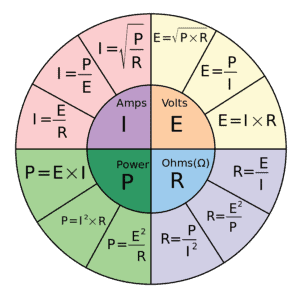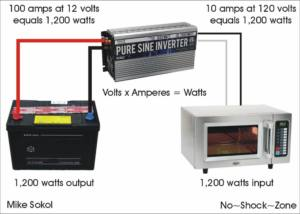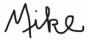Monday, December 4, 2023

# RVelectricity: Can I make more power by stepping up the voltage?

By Mike SokolDear Mike,
After reading some of your articles about voltage from the electric company, I see there could be up to 10,000 volts or more of power coming to the transformer on the power pole, which then gets stepped down to the 120 volts we need for our homes and RVs. If that’s the case, why can’t I just step it back up to 10,000 volts with a transformer to make more power? Or am I missing something important? —Steve

Dear Steve,
Great question, which allows me to discuss Watt’s law as it relates to power. And what you’re confused about in your thought process is “power.” That’s right – voltage isn’t power at all. Watts is power, and more specifically watt-hours is a measurement of energy. Let me explain.As I just hinted, the only thing that really matters is the power available to run anything, be it an air conditioner, electric space heater, radio or even your electric toothbrush. And in its simplest form, electric power is measured in watts. If you take a quick look at the combined Ohm’s and Watt’s law wheel you can see that Watts (P for Power) is defined as Volts (E for Electromotive Force) times Amperes (I for Intensity of Current). Written simply it looks like E x I = P (Volts times Amps equals Watts).

So let’s assume you have a 20-amp circuit breaker on your 120-volt AC connection. To find out how many watts are available you just multiple 20 amps times 120 volts and get 2,400 watts. All good so far, correct?

Now what if you were to run it into a 100:1 ratio transformer to step up the voltage by a factor of 100? That’s easy enough to calculate since 100 times 120 volts is equal to 12,000 volts (100 x 120 = 12,000). But we then need to flip the equation around to find out how many amperes of current are available. So we need to run P/E=I. If you divide 2,400 watts by 12,000 volts we’ll find that there’s only 0.2 amperes of current available. What’s happening is you’re trading volts for amps. If everything else remains equal, then multiplying the voltage by 100 divides the current by 100.The same basic process happens in your house battery and inverter. Watts is watts, so if you need 1,200 watts at 120 volts that’s going to calculate at 1,200 watts / 120 volts = 10 amperes. But on the 12-volt battery side we need to calculate this as 1,200 watts / 12 volts = 100 amperes.

In both cases we’re just stepping the voltage up or down by a factor of 10, and the current does the opposite thing in the same ratio. So if you boost the voltage by 10 times, you reduce the current by the same factor of 10, and visa versa. Since 12-volt batteries and 120-volt AC are a convenient 10:1 ratio (more or less), you just multiply or divide by 10 to find out how much current is flowing in or out of your RV batteries. So if you need to charge your batteries with 60 amperes of current at 12 volts, that’s only 6 amperes of current needed at 120 volts AC.

Sorry Steve, but there’s no such thing as a free lunch (TNSTAAFL). Energy can only be converted into other forms, not created or destroyed. Next time we’ll discuss how to turn those watts of power into watt-hours of energy. See you then.

Let’s play safe out there….Mike Sokol is an electrical and professional sound expert with 50+ years in the industry. His excellent book RV Electrical Safety is available at Amazon.com. For more info on Mike’s qualifications as an electrical expert, click here.

For information on how to support RVelectricity and No~Shock~Zone articles, seminars and videos, please click the I Like Mike Campaign.

##RVT961

Article Rating
Notify of

This site uses Akismet to reduce spam. Learn how your comment data is processed.

Inline Feedbacks

Keira B (@guest_91650)
3 years ago

Since you are giving us a lesson in basic electricity, we should probably be technically accurate.
Ohm’s law is E = R * I. The equation does not involve power or energy. Only Voltage (E), Resistance (R), and Current (I).
The Power Law equation is P = E * I.
Also, Watts are the measurement of power in the common American usage. Not the same as Watt-Hours, which is a unit of energy.

John T (@guest_91369)
3 years ago

Watt-hours is a measurement of energy, not power.
1 watt-hour = 3600 watt-second = 3600 joule.

3 years ago

Great explanation for all of us non-electrically minded folks. Thanks for answering this question so the we can understand this all.

Jim Stein (@guest_91260)
3 years ago

This may be the best explanation of Ohm’s Law that I have seen. Many times I have tried to convey this to the blank stares of friends and family. From now on, I’m going to just print this out and hand it to people. Well done.